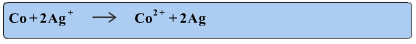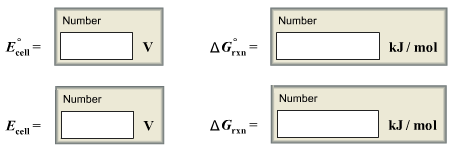# Problem: For the following electrochemical cellCo(s) | Co2+ (aq, 0.0155 M) || Ag+ (aq, 1.50 M) | Ag(s)Write the net cell equation. Phases are optional. Do not include the concentrations.Calculate the following values at 25.0°C using standard potentials as needed.

###### FREE Expert Solution

For a Cobalt-Silver cell with the given diagram, we’re asked to write the net cell equation and to calculate E°cell and Δrxn at 25 °C.

The cell diagram of the process is:

Co(s) | Co2+ (aq, 0.0155 M) || Ag+ (aq, 1.50 M) | Ag(s)

Where

Co(s) is the left-hand electrode

Co2+ is the left-hand cation in the aqueous electrolyte

Ag+ is the right-hand cation in the aqueous electrolyte

Ag is the right-hand electrode

There are two ways to identify the Cathode and Anode:

• The Cathode is related to the reduction process, and the Anode is related to the Oxidation process.
• On the cell diagram, the / symbol indicates the phase boundaries or the different phases of an element, while the // symbol indicates the physical boundary between cells. The first phase boundary is our Anode (A), the second the Cathode (C), and the physical boundary is our Break (B).

A                                 B                           C

Co(s) | Co2+ (aq, 0.0155 M) || Ag+ (aq, 1.50 M) | Ag(s)

We answer the problem in 5 steps:

Step 1: Balance the charges: add electrons to the more positive side (or less negative side)

Step 2: Balance electrons on the two half-reactions.

92% (180 ratings)###### Problem Details

For the following electrochemical cell

Co(s) | Co2+ (aq, 0.0155 M) || Ag+ (aq, 1.50 M) | Ag(s)

Write the net cell equation. Phases are optional. Do not include the concentrations.Calculate the following values at 25.0°C using standard potentials as needed.